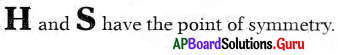# AP Board 7th Class Maths Solutions Chapter 12 Symmetry Ex 12.3

SCERT AP 7th Class Maths Solutions Pdf Chapter 12 Symmetry Ex 12.3 Textbook Exercise Questions and Answers.

## AP State Syllabus 7th Class Maths Solutions 12th Lesson Symmetry Ex 12.3

Question 1.
Draw lines of symmetry for the following figures. Identify which of them have point symmetry. Is there any relation between lines of symmetry and point symmetry?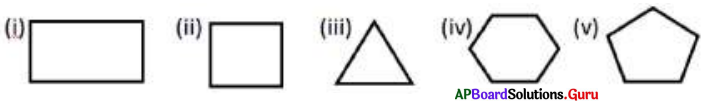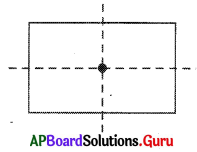(i) Rectangle has point of symmetry.
Number of lines of symmetry = 2
Order of symmetry = 2
Lines of symmetry point or symmetry are same.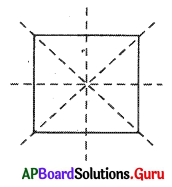(ii) Square has a point of symmetry.
Number of lines of symmetry = 4
Order of symmetry = 4
Lines of symmetry, point of symmetry are same.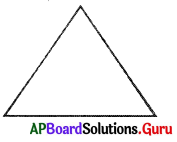(iii) Triangle has no point of symmetry.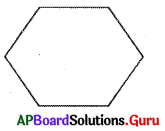(iv) Regular hexagon has point of syrninetìy.
Number of lines of symmetry = 6
Order of symmetry = 6
Lines of symmetry point of symmetry are same.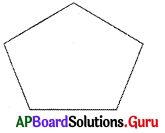(v) Pentagon has no point of symnetryQuestion 2.
Identify which of the English alphabet have point symmetry in the following: